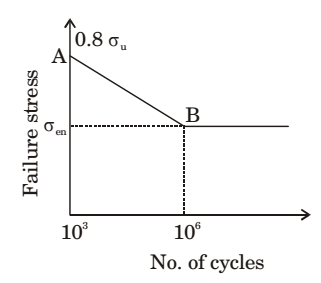## Machine Design Miscellaneous

#### Machine Design

1. A 60 mm long and 6 mm thick fillet weld carries a steady load of 15 kN along the weld. The shear strength of the weld material is equal to 200 MPa. The factor of safety is

1. P = .707 t × Le × τs.

 15 × 103 = τs .707 × 6 × 60

∴ τs = 58.94 MPa
Given shear strength of material = 200 MPa
 FOS = 200 = 3.4 58.94

##### Correct Option: B

P = .707 t × Le × τs.

 15 × 103 = τs .707 × 6 × 60

∴ τs = 58.94 MPa
Given shear strength of material = 200 MPa
 FOS = 200 = 3.4 58.94

1. Endurance limit of a beam subjected to pure bending decreases with

1. NA

##### Correct Option: A

NA

1. Fatigue life of a material for a fully reversed loading condition is estimated from σa = 1100 N–0.15 where σa is the stress amplitude in MPa and N is the failure life in cycles. The maximum allowable stress amplitude (in MPa) for a life of 1 × 105 cycles under the same loading condition is_______(correction to two decimal places).

1.  σmax - σmin = 1100N-0.15 2

 σmax - (- σmin) = 1100N-0.15 2

 2σmax = 1100N-0.15(N = 105) 2

∴ We get, σmax = 195.61 N

##### Correct Option: A

 σmax - σmin = 1100N-0.15 2

 σmax - (- σmin) = 1100N-0.15 2

 2σmax = 1100N-0.15(N = 105) 2

∴ We get, σmax = 195.61 N

1. A machine element has an ultimate strength (σu) of 600 N/mm², and endurance limit (σen) of 250 N/mm². The fatigue curve for the element on a log-log plot is shown below. If the element is to be designed for a finite life of 10000 cycles, the maximum amplitude of a completely reversed operating stress is ______N/mm².1.  log Sf - log (0.8 × 600) = 4 - 3 log 250 - log (0.6 × 600) 6 - 3

 ⇒ log Sf - log (480) = 1 log 250 - log (360) 3

Sf = 386.19 MPa

##### Correct Option: A

 log Sf - log (0.8 × 600) = 4 - 3 log 250 - log (0.6 × 600) 6 - 3

 ⇒ log Sf - log (480) = 1 log 250 - log (360) 3

Sf = 386.19 MPa

1. At a critical point in a component, the state of stress is given as σxx = 100 MPa, σyy = 220 MPa, σxy = σyx = 80 M Pa and al l ot her st r ess components are zero. The yield strength of the material is 468 MPa. The factor of safety on the basis of maximum shear stress theory is_____ (round off to one decimal place).

1. Given, σx = 100 MPa
σy = 220 MPa
σxy = 80 MPa= 160 ± √55² + 80²
σ1, 2 = 160 ± 97.0824
⇒ σ1 = 257.08 Mpa, σ2 = 62.9 Mpa
As per maximum shear stress theorySyt = σ1 = 257.08 2N 2 2

 N = 468 = 1.8 257.08

##### Correct Option: A

Given, σx = 100 MPa
σy = 220 MPa
σxy = 80 MPa= 160 ± √55² + 80²
σ1, 2 = 160 ± 97.0824
⇒ σ1 = 257.08 Mpa, σ2 = 62.9 Mpa
As per maximum shear stress theorySyt = σ1 = 257.08 2N 2 2

 N = 468 = 1.8 257.08